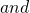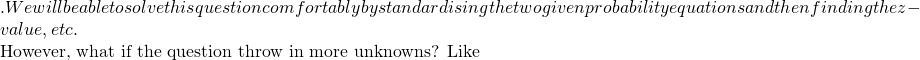# Using symmetrical properties of Normal Curve to solve questionsConsider if we are simply given thatlatex \mathrm{P}(X < 4.2) = 0.2latex \mathrm{P}(X > 8) = 0.1latex \alpha \mathrm{P}(X < \mu_0 – \alpha) = \mathrm{P}(X < \mu_0 + \alpha)latex \mu\$. And it so happens that the mean of the standard normal distribution is zero.

Students may want to reference a 2014 ACJC Prelim Question here to see if they can do it.

Not readable? Change text.